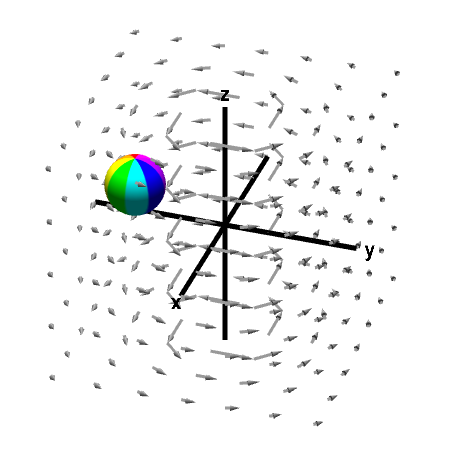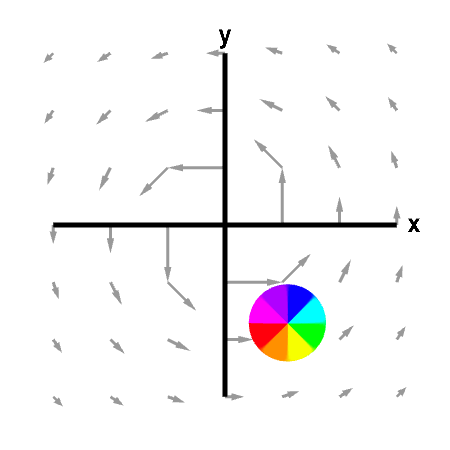# Math Insight

### Applet: Circling sphere in a vector field with zero curlThe sphere is circling around the $z$-axis due to the macroscopic circulation of the vector field. However, away from the $z$-axis, the vector field has no microscopic circulation, i.e., its curl is zero. This example illustrates that one cannot infer curl from the macroscopic circulation of the vector field. Macroscopic and microscopic circulation can be very different. First panel shows the full vector field; second panel shows its projection in the $xy$-plane. You can rotate the first panel with the mouse to better visualize the three-dimensional perspective.

This vector field is $$\dlvf(x,y,z) = \frac{(-y,x,0)}{x^2+y^2}$$ for $(x,y) \ne (0,0)$. Since for $(x,y) \ne (0,0)$, $$\pdiff{\dlvfc_2}{x} =\pdiff{\dlvfc_1}{y}= \frac{y^2-x^2}{(x^2+y^2)^2},$$ one can compute that away from the $z$-axis, $\curl \dlvf(x,y,z) = (0,0,0)$.